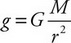# AP Physics 1 Question 118: Answer and Explanation

### Test Information

Question: 118

8. The radius of Mars is about half that of Earth; the mass of Mars is about one-tenth that of Earth. Which of the following is closest to the gravitational field at the surface of Mars?

• A. 10 N/kg
• B. 4 N/kg
• C. 2 N/kg
• D. 0.5 N/kg

B-The gravitational field at the surface of a planet is. The numerator for Mars is 1/10 that of Earth, reducing the gravitational field by 1/10. The denominator for Mars is (1/2)2 = 1/4 that of Earth, increasing the gravitational field by a factor of 4 (because a smaller denominator means a bigger fraction). The overall gravitational field is multiplied by 4/10. On Earth, the gravitational field is 10 N/kg, so on Mars, g = 4 N/kg.